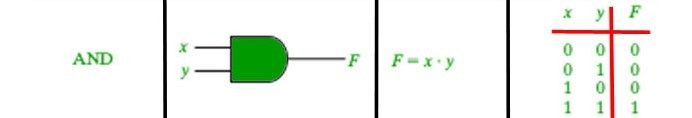# && operator in Java with Examples

&& is a type of Logical Operator and is read as “AND AND” or “Logical AND“. This operator is used to perform “logical AND” operation, i.e. the function similar to AND gate in digital electronics.One thing to keep in mind is the second condition is not evaluated if the first one is false, i.e. it has a short-circuiting effect. Used extensively to test for several conditions for making a decision.

Syntax:

```Condition1 && Condition2

// returns true if both the conditions are true.
```

Below is an example to demonstrate && operator:

Example:

 `// Java program to illustrate ` `// logical AND operator ` ` `  `import` `java.util.*; ` ` `  `public` `class` `operators { ` `    ``public` `static` `void` `main(String[] args) ` `    ``{ ` ` `  `        ``int` `num1 = ``10``; ` `        ``int` `num2 = ``20``; ` `        ``int` `num3 = ``30``; ` ` `  `        ``// find the largest number ` `        ``// using && operator ` `        ``if` `(num1 >= num2 && num1 >= num3) ` `            ``System.out.println( ` `                ``num1 ` `                ``+ ``" is the largest number."``); ` `        ``else` `if` `(num2 >= num1 && num2 >= num3) ` `            ``System.out.println( ` `                ``num2 ` `                ``+ ``" is the largest number."``); ` `        ``else` `            ``System.out.println( ` `                ``num3 ` `                ``+ ``" is the largest number."``); ` `    ``} ` `} `

Output:

```30 is the largest number.
```
Whether you're preparing for your first job interview or aiming to upskill in this ever-evolving tech landscape, GeeksforGeeks Courses are your key to success. We provide top-quality content at affordable prices, all geared towards accelerating your growth in a time-bound manner. Join the millions we've already empowered, and we're here to do the same for you. Don't miss out - check it out now!

Previous
Next